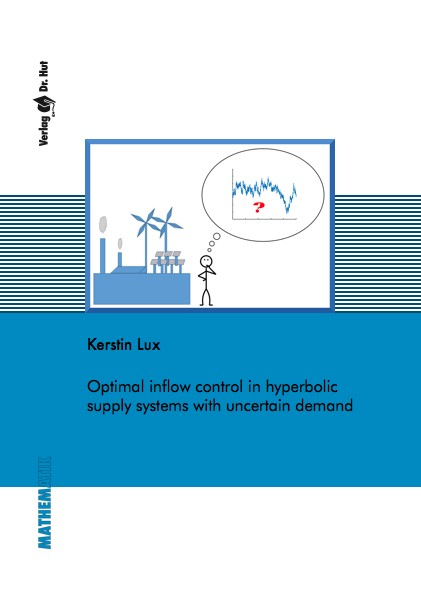Datenbestand vom 16. September 2021aktualisiert am 16. September 2021

# ISBN 978-3-8439-4479-345,00 € inkl. MwSt, zzgl. Versand

978-3-8439-4479-3, Reihe Mathematik

Kerstin Lux
Optimal inflow control in hyperbolic supply systems with uncertain demand

207 Seiten, Dissertation Universität Mannheim (2020), Softcover, A5

## Zusammenfassung / Abstract

In this thesis, we investigate the optimal inflow control in hyperbolic supply systems with uncertain demand. Taking uncertainty into account becomes more and more important in many areas such as production planning and energy grid operation. Deviations from the demand actually realized need to be compensated, which might be very costly and should be avoided. This thesis provides a stochastic optimal control framework to cope with an uncertain demand stream.

We consider different control methods for the inflow in a hyperbolic supply system. These methods allow a distinction between different information levels about the demand available to the controller.

The hyperbolic supply system is modeled by a hyperbolic conservation respectively balance law. This type of equation belongs to the mathematical area of hyperbolic partial differential equations (PDEs) and describes the flow of quantities of interest within the supply system. The uncertain demand stream is modeled as a stochastic process. We present results using the Ornstein-Uhlenbeck process with time-dependent mean reversion level and a jump diffusion process.

We develop different solution approaches for the stochastic optimal control problem. For a linear transportation process, we derive its analytical solution. To take into account more complex flow dynamics, we come up with alternative solution approaches that are based on a deterministic reformulation of the originally stochastic optimal control problem. This is beneficial as well-known methods from deterministic PDE-constrained optimization can be applied. We analyze these approaches both from a theoretical as well as from a numerical point of view.

To enhance supply reliability, we mathematically formulate the requirement of demand satisfaction at a prescribed probability level in terms of two types of chance constraints. A reformulation of the probabilistic constraints allows their inclusion into the stochastic optimal control framework.